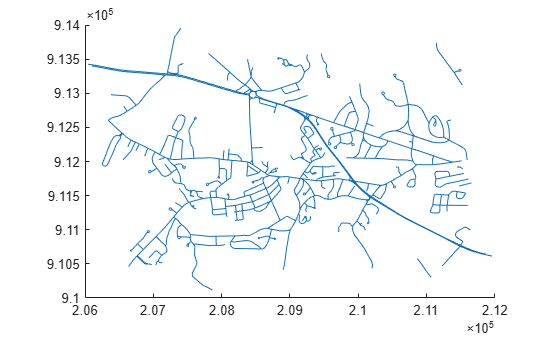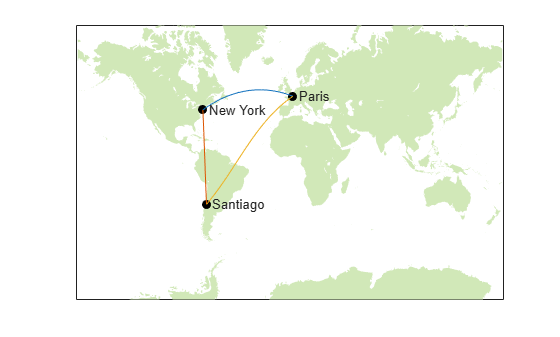## Geographic Data Structures

Vector data uses points, lines, polygons, and their nonspatial attributes to describe events, locations, and objects. One way to represent vector data is by using geographic data structures. A geographic data structure is a structure array that contains one element per geographic feature.

There are two types of geographic data structures. A mapstruct array stores map coordinates (x and y) using `X` and `Y` fields, and a geostruct array stores geographic coordinates (latitude and longitude) using `Lon` and `Lat` fields.

Note

Starting in R2021b, you can also represent vector data by using geospatial tables. Geospatial tables are `table` or `timetable` objects with a `Shape` variable and attribute variables. Geospatial tables enable you to:

There are several ways to create geographic data structures:

• Read a geographic data structure from a shapefile by using the `shaperead` function.

• Read a geographic data structure from GSHHG data by using the `gshhs` function.

• Create a geographic data structure from variables in the workspace.

### Fields of Geographic Data Structures

Geographic data structures contain these fields:

FieldDescription
`Geometry`

Type of shape, specified as a character vector such as `'Point'`, `'Multipoint'`, `'Line'`, or `'Polygon'`.

Individual points, lines, and polygons can have multiple parts. For example, lines can contain disconnected line segments, and polygons can have rings. All parts of an individual shape share the attributes specified for that shape by the corresponding attribute fields. Geographic data structures do not support assigning distinct attributes to the different parts of an individual shape.

`BoundingBox`

Minimum and maximum coordinate values for the shape, specified as a 2-by-2 numeric matrix of the form `[min(X) min(Y); max(X) max(Y)]`. When `Geometry` is `'Point'`, this field does not apply.

`X`

x-coordinates, specified as a row vector of data type `double`. This field applies to only mapstruct arrays.

`Y`

y-coordinates, specified as a row vector of data type `double`. This field applies to only mapstruct arrays.

`Lon`

Longitude coordinates, specified as a row vector of data type `double`. This field applies to only geostruct arrays.

`Lat`

Latitude coordinates, specified as a row vector of data type `double`. This field applies to only geostruct arrays.

Attribute fields

Attribute fields, specified as character vectors or numeric scalars.

### Read Geographic Data Structures from Shapefiles

This example shows how to read data from a shapefile into a geographic data structure by using the `shaperead` function. The `shaperead` function is the most common way to create geographic data structures.

Starting in R2021b, you can read the same data into a geospatial table by using the `readgeotable` function. The `readgeotable` function supports several vector data formats in addition to shapefiles, including KML and GeoJSON files.

Read a shapefile containing road data into the workspace. The function returns a geographic data structure (in this case a mapstruct array) that contains 609 elements. In addition to the `Geometry`, `BoundingBox`, and coordinate fields (`X` and `Y`), the mapstruct array has five attribute fields: `STREETNAME`, `RT_NUMBER`, `CLASS`, `ADMIN_TYPE`, and `LENGTH`.

`S = shaperead("concord_roads.shp")`
```S=609×1 struct array with fields: Geometry BoundingBox X Y STREETNAME RT_NUMBER CLASS ADMIN_TYPE LENGTH ```

Query the 10th element of the mapstruct array.

`S(10)`
```ans = struct with fields: Geometry: 'Line' BoundingBox: [2x2 double] X: [2.0892e+05 2.0892e+05 2.0893e+05 2.0894e+05 2.0895e+05 2.0896e+05 2.0897e+05 2.0898e+05 NaN] Y: [9.1342e+05 9.1341e+05 913405 9.1340e+05 9.1340e+05 9.1340e+05 913407 9.1342e+05 NaN] STREETNAME: 'WRIGHT FARM' RT_NUMBER: '' CLASS: 5 ADMIN_TYPE: 0 LENGTH: 79.0347 ```

Display the road data on a map.

`mapshow(S)`By default, the `shaperead` function assumes that the shapefile contains map coordinates and reads the data into a mapstruct array. When your shapefile contains geographic coordinates, you can read the data into a geostruct array by specifying the `UseGeoCoords` name-value argument as `true`. If you do not know whether a shapefile contains map coordinates or geographic coordinates, you can try these strategies.

• If the shapefile includes a projection file (`.prj`), you can get information about the coordinate reference system by using the `shapeinfo` function. If the `CoordinateReferenceSystem` field of the returned structure is a `projcrs` object, then the shapefile contains map coordinates. If the field is a `geocrs` object, then the shapefile contains geographic coordinates.

• If the shapefile does not include a projection file, you can get the bounding box of the coordinates by using the `shapeinfo` function. You might be able to determine the type of coordinates by looking at the ranges of the coordinates.

If you cannot determine whether your shapefile contains map coordinates or geographic coordinates using these strategies, consider asking your data provider.

### Create Geographic Data Structures

Create geographic data structures from variables in the workspace. The variables can represent data imported from files or data computed using MATLAB® functions.

Starting in R2021b, you can also create geospatial tables from variables in the workspace. For more information about creating geospatial tables, see Create Geospatial Tables.

Create Geostruct Array of Points

Create a geostruct array of points. For this example, use the geostruct array to store the locations and names of cities.

Specify the latitude and longitude coordinates of Paris, Santiago, and New York City.

```latP = 48.87084; lonP = 2.41306; latS = -33.36907; lonS = -70.82851; latN = 40.69746; lonN = -73.93008; ```

Create an empty structure array. Add a `Geometry` field that defines the structure array elements as points. Add `Lat` and `Lon` fields that store the coordinates of the points.

```Cities = struct; [Cities(1:3).Geometry] = deal('Point'); Cities(1).Lat = latP; Cities(1).Lon = lonP; Cities(2).Lat = latS; Cities(2).Lon = lonS; Cities(3).Lat = latN; Cities(3).Lon = lonN;```

Add a `Name` attribute field that stores the names of the cities.

```Cities(1).Name = "Paris"; Cities(2).Name = "Santiago"; Cities(3).Name = "New York";```

View the geostruct array.

`Cities`
```Cities=1×3 struct array with fields: Geometry Lat Lon Name ```

Create Geostruct Array of Lines

Create a geostruct array of lines. For this example, use the geostruct array to store great circle tracks between the cities.

Create an empty structure array. Add a `Geometry` field that defines the structure array elements as lines.

```Tracks = struct; [Tracks(1:3).Geometry] = deal('Line');```

Add a `Type` attribute field that identifies the tracks as great circle tracks. Many functions use the input `"gc"` to identify tracks as great circle tracks.

```trackType = "gc"; [Tracks.Type] = deal(trackType);```

Calculate the coordinates of the great circle tracks between the cities. Then, add the coordinates to the structure array as `Lat` and `Lon` fields.

```[latPS,lonPS] = track2(trackType,latP,lonP,latS,lonS); [latSN,lonSN] = track2(trackType,latS,lonS,latN,lonN); [latNP,lonNP] = track2(trackType,latN,lonN,latP,lonP); Tracks(1).Lat = latPS; Tracks(1).Lon = lonPS; Tracks(2).Lat = latSN; Tracks(2).Lon = lonSN; Tracks(3).Lat = latNP; Tracks(3).Lon = lonNP;```

Label the track names by adding a `Name` attribute field to the structure array.

```Tracks(1).Name = "Paris-Santiago"; Tracks(2).Name = "Santiago-New York"; Tracks(3).Name = "New York-Paris";```

Calculate the lengths and azimuths of the great circle tracks. Then, add the results to the structure array as `Length` and `Azimuth` attribute fields.

```[distPS,azPS] = distance(trackType,latP,lonP,latS,lonS); [distSN,azSN] = distance(trackType,latS,lonS,latN,lonN); [distNP,azNP] = distance(trackType,latN,lonN,latP,lonP); Tracks(1).Length = distPS; Tracks(1).Azimuth = azPS; Tracks(2).Length = distSN; Tracks(2).Azimuth = azSN; Tracks(3).Length = distNP; Tracks(3).Azimuth = azNP;```

View the geostruct array.

`Tracks`
```Tracks=1×3 struct array with fields: Geometry Type Lat Lon Name Length Azimuth ```

Display Data Contained in Geostruct Arrays

Display the city locations and names on the map.

```figure axesm("mercator","MapLatLimit",[-75 75]) tightmap geoshow("landareas.shp","FaceColor",[0.82 0.91 0.72],"EdgeColor","none") geoshow(Cities,"Marker","o","MarkerFaceColor","k","MarkerEdgeColor","k") textm([Cities.Lat],[Cities(:).Lon]+5,[Cities(:).Name])```

Display the great circle tracks on the map. Specify the appearance of the lines by using a symbol specification.

```colorRange = makesymbolspec("Line",{'Length',[min([Tracks.Length]) max([Tracks.Length])],"Color",lines(3)}); geoshow(Tracks,"SymbolSpec",colorRange)```Create Geostruct Array of Polygons

The process for creating a geostruct or mapstruct array of polygons is similar to the process for creating geostruct arrays of points and lines. For information about specifying the coordinates of polygon vertices, see Create and Display Polygons.

For an example of working with geostruct arrays of polygons, see Converting Coastline Data (GSHHG) to Shapefile Format.